## Abstract

A computational study has been performed to make a computational analysis of natural convection and entropy generation in a sharp edged finned cavity. Three dimensional analysis has been done by solving governing equations with a written computational code in Fortran. The study is performed for fin inclination angles from −60° to 60°, Ra = 105, Pr = 0.7, Rc   (conductivities ratio) changes from 0.01 to 100 and irreversibility coefficient is taken as ${\textstyle \varphi ={10}^{-5}}$. It is observed that higher values of thermal conductivity ratio (Rc ⩾ 1) do not affect entropy generation due to heat transfer and Bejan number.

## Keywords

Entropy generation; Inclined fin; Natural convection; Three dimensional

## Nomenclature

Be- Bejan number

Cp- specific heat at constant pressure (J/kg K)

g- gravitational acceleration (m/s2)

k- thermal conductivity (W/m K)

l- cavity width

n- unit vector normal to the wall

Ns- dimensionless local generated entropy

Nu- local Nusselt number

Pr- Prandtl number

Ra- Rayleigh number

Rc- thermal conductivity ratio

${\textstyle S_{gen}^{'}}$- generated entropy (kJ/kg K)

t- dimensionless time ${\textstyle \left(t^{'}\cdot \alpha /l^{2}\right)}$

T- dimensionless temperature ${\textstyle \left[(T^{'}-T_{c}^{'})/(T_{h}^{'}-T_{c}^{'})\right]}$

${\textstyle T_{c}^{'}}$- cold temperature (K)

${\textstyle T_{h}^{'}}$- hot temperature (K)

To- bulk temperature ${\textstyle \left[T_{o}=(T_{c}^{'}+T_{h}^{'})/2\right]}$ (K)

${\textstyle {\overset {\rightarrow }{V}}}$- dimensionless velocity vector ${\textstyle \left({\overset {\rightarrow }{V}}^{'}\cdot l/\alpha \right)}$

x, y, z- dimensionless Cartesian coordinates ${\textstyle \left(x^{'}/l{\mbox{,}}y^{'}/l{\mbox{,}}z^{'}/l\right)}$

### Greek symbols

${\textstyle \alpha }$- thermal diffusivity (m2/s)

${\textstyle \beta }$- thermal expansion coefficient (1/K)

${\textstyle \varphi }$- irreversibility coefficient

${\textstyle \theta }$- fin inclination

${\textstyle \rho }$- density (kg/m3)

${\textstyle \mu }$- dynamic viscosity (kg/m s)

${\textstyle \nu }$- kinematic viscosity (m2/s)

${\textstyle {\overset {\rightarrow }{\psi }}}$- dimensionless vector potential (${\textstyle {\overset {\rightarrow }{\psi }}^{'}/\alpha }$)

${\textstyle {\overset {\rightarrow }{\omega }}}$- dimensionless vorticity ${\textstyle \left({\overset {\rightarrow }{\omega }}^{'}\cdot \alpha /l^{2}\right)}$

${\textstyle \Delta T}$- dimensionless temperature difference

### Subscripts

av- average

x, y, z- Cartesian coordinates

fr- friction

f- fluid

av- average

nf- nanofluid

s- solid

th- thermal

tot- total

### Superscript

′- dimensional variable

## 1. Introduction

Natural convection heat transfer occurs in many field of engineering such as cooling of electronic equipment, solar collectors, heating of buildings, heat exchangers and many other applications. Control of energy is extremely important to save energy consumption. Thus, entropy generation analysis is highly important to answer the question of why or where energy is consumed in the system?

Using of passive systems such as baffle, fin or obstacle is a simple way to control heat and fluid flow due to natural convection in cavities. Varol et al.  studied the laminar natural convection heat transfer in an inclined fin located cavity by solving two dimensional governing equations. Also, they performed an experiment to compare their results and found the inclination angle of the fin is the important parameter to control heat and fluid flow. As a similar work, Ozgen et al.  tested the effects of Prandtl number and baffle location on natural convection. Li and Byon  studied the orientation effect on the thermal performance of radial heat sinks with a circular base, concentric ring, and rectangular fins both experimentally and numerically. They observed that the radial heat sink in orientation 0 is found to have slightly better thermal performance than that of 180. Saravanan and Sivaraj  performed a numerical work on combined natural convection and thermal radiation in a square cavity with a non-uniformly heated plate. Effects of heat generating baffles on natural convection in a square cavity were studied by Saravanan and Vidhya Kumar . In their study, the baffles were kept centrally separated by a distance d in the cavity and assumed to generate heat uniformly. They observed that flow inhibition mechanism was dominating only when the baffles are away from the cavity walls. Other related works on two-dimensional analysis of natural convection in inclined fin attached cavities are studied in Refs. , ,  and .

Importance of analyses of entropy generation from the energy efficiency point of view is presented in reviewable work of Öztop and Al-Salem  and Sciacovelli et al. . They mainly focused on entropy generation in natural and mixed convection heat transfer. Shuja et al.  solved a problem on natural convection in a square cavity with a heat generating body by considering entropy generation. Khorasanizadeh et al.  performed a numerical study of natural convection and entropy generation of Cu-water nanofluid within an enclosure with a conductive baffle embedded on bottom hot wall. They solved the governing equations numerically with Finite Volume Method by using the SIMPLER algorithm. They showed that assuming a constant irreversibility factor, χ, with change of Ra and ϕ is not correct.

Entropy generation due to natural convection in an enclosure heated locally from below with two isoflux sources was investigated by Mukhopadhyay . They studied unequal heater length and heater strength, the effects of heater length and strength ratios. It is found that minimum entropy generation rate was achieved for the same condition at which the minimum peak heater temperature was obtained. Famouri and Hooman  studied the entropy generation in two-dimensional domain to investigate the entropy generation for natural convection by heated partitions in a cavity.

Dalir  studied numerically the entropy generated by laminar forced convection flow and heat transfer of an incompressible Jeffrey non-Newtonian fluid over a linearly stretching, impermeable and isothermal sheet. Results indicate that the generated entropy increases with the increase in Deborah number and decreases by the increase in ratio of relaxation to retardation times. Das et al.  investigated the entropy generation in a MHD pseudo-plastic nanofluid flow through a porous channel with convective heating. Three different types of nanoparticles were considered and several parameters were varied to find the minimum of entropy generation.

Studies on three dimensional domain of natural convection are very limited. In this context, Wakashima and Saitoh  and Fusegi et al.  made studies to analyze the natural convection in three dimensional domains.

The main aim of this work was to study the effects of presence of inclined fin on natural convection heat transfer fluid flow and heat transfer in a three dimensional domain of cavity. Entropy generation is calculated by using obtained data of velocity and temperature. Entropy generation due to heat transfer, fluid flow and total entropy generation are presented via contours and variation according to thermal conductivity ratio.

## 2. Physical model

The considered physical model is presented in Fig. 1(a) (on the left) and Fig. 1(b) (on the right). As seen from the figure, the cavity is heated from the bottom wall and cooled from the top while vertical walls are adiabatic. A sharp edged fin is located to left vertical wall to control heat and fluid flow. This fin is conductive and its conductivity changed from Rc = 0.01 to 100. Inclination angle of the fin changes from −60° to 60° and thickness and length of the fin are taken as constant.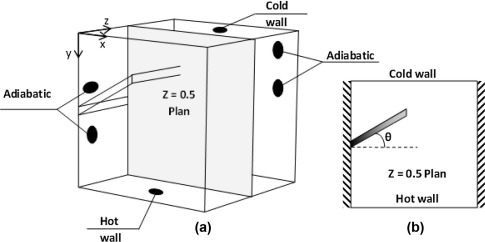Figure 1. (a) Three dimensional physical model, and (b) two-dimensional view.

## 3. Computational study

### 3.1. Governing equations

The 3D vorticity-vector potential formalism ${\textstyle \left({\overset {\rightarrow }{\psi }}-{\overset {\rightarrow }{\omega }}\right)}$ is used as numerical method, in order to eliminate the pressure term, which is delicate to treat. ${\textstyle \left({\overset {\rightarrow }{\psi }}\right)}$ and ${\textstyle \left({\overset {\rightarrow }{\omega }}\right)}$ are respectively defined by the two following relations:

 ${\overset {\rightarrow }{\omega }}^{'}={\overset {\rightarrow }{\nabla }}\times {\overset {\rightarrow }{u^{'}}}\quad {\mbox{and}}\quad {\overset {\rightarrow }{u^{'}}}=$${\overset {\rightarrow }{\nabla }}\times {\overset {\rightarrow }{\psi }}^{'}$
(1)

After non-dimensionalization the system of equations controlling the phenomenon is as follows:

 $-{\overset {\rightarrow }{\omega }}={\nabla }^{2}{\overset {\rightarrow }{\psi }}$
(2)

 ${\frac {\partial {\overset {\rightarrow }{\omega }}}{\partial t}}+$$({\overset {\rightarrow }{u}}\cdot \nabla ){\overset {\rightarrow }{\omega }}-$$({\overset {\rightarrow }{\omega }}\cdot \nabla ){\overset {\rightarrow }{u}}\cdot =$$\Delta {\overset {\rightarrow }{\omega }}+{\mbox{Ra}}\cdot Pr\cdot \left[{\frac {\partial T}{\partial z}}{\mbox{,}}-\right.$$\left.{\frac {\partial T}{\partial x}}{\mbox{,}}0\right]$
(3)

 ${\begin{array}{l}{\frac {\partial T}{\partial t}}+{\overset {\rightarrow }{u}}\cdot \nabla T={\nabla }^{2}T\quad {\mbox{in fluid}}\\{\frac {\partial T}{\partial t}}={\frac {{\alpha }_{s}}{{\alpha }_{f}}}{\nabla }^{2}T\quad {\mbox{in solid}}\end{array}}$
(4)
 ${\mbox{with}}:\quad Pr={\frac {\nu }{{\alpha }_{f}}}{\mbox{,}}\quad {\mbox{Ra}}=$${\frac {g{\beta }_{T}(T_{h}-T_{c})l^{3}}{\nu \cdot {\alpha }_{f}}}$

The energy equation (conduction) needs to be solved in the solid portion of the domain, and the fin conductivity ks is assumed constant. At the solid–fluid interface the temperature and heat flux must be continuous. The latter requirement is mathematically expressed as

 ${\left({\frac {\partial T}{\partial n}}\right)}_{f}=R_{c}{\left({\frac {\partial T}{\partial n}}\right)}_{s}$
(5)

where ${\textstyle R_{c}=k_{s}/k_{f}}$ is the thermal conductivity ratio between the material of the fin and the medium that fills the cavity. There is no flow in the fraction of the volume occupied by the blinds (solid). The control volume finite difference method is used to discretize Eqs. (1), (2), (3) and (4). The central-difference scheme for treating convective terms and the fully implicit procedure to discretize the temporal derivatives are retained. The grid is uniform in all directions with additional nodes on boundaries. The successive relaxation iterating scheme is used to solve the resulting nonlinear algebraic equations. The time step 10−4 and spatial mesh 81 × 81 × 81 are retained to carry out all numerical tests. The solution is considered acceptable when the following convergence criterion is satisfied for each step of time as

 $\sum _{i}^{1{\mbox{,}}2{\mbox{,}}3}{\frac {max\vert {\psi }_{i}^{n}-{\psi }_{i}^{n-1}\vert }{max\vert {\psi }_{i}^{n}\vert }}+$$max\vert T_{i}^{n}-T_{i}^{n-1}\vert \leqslant {10}^{-5}$
(6)

Boundary conditions for considered model are given as follows:

Temperature boundary conditions

 $T=0\quad {\mbox{for}}\quad \quad y=1\quad {\mbox{and}}\quad T=$$1\quad {\mbox{for}}\quad y=0{\mbox{,}}$

${\textstyle {\frac {\partial T}{\partial n}}=0}$ on all other walls (adiabatic).

Vorticity

 ${\begin{array}{rl}&{\omega }_{x}=0{\mbox{,}}{\omega }_{y}=-{\frac {\partial u_{z}}{\partial x}}{\mbox{,}}{\omega }_{z}={\frac {\partial u_{y}}{\partial x}}\quad {\mbox{at}}\quad x=0\quad {\mbox{and}}\quad \quad 1\\&{\omega }_{x}={\frac {\partial u_{z}}{\partial y}}{\mbox{,}}{\omega }_{y}=0{\mbox{,}}{\omega }_{z}=-{\frac {\partial u_{x}}{\partial y}}\quad {\mbox{at}}\quad y=0\quad {\mbox{and}}\quad 1\\&{\omega }_{x}=-{\frac {\partial u_{y}}{\partial z}}{\mbox{,}}{\omega }_{y}={\frac {\partial u_{x}}{\partial z}}{\mbox{,}}{\omega }_{z}=0\quad {\mbox{at}}\quad z=0\quad {\mbox{and}}\quad 1\end{array}}$

Vector potential

 ${\begin{array}{rl}&{\frac {\partial {\Psi }_{x}}{\partial x}}={\Psi }_{y}={\Psi }_{z}=0\quad {\mbox{at}}\quad x=0\quad {\mbox{and}}\quad 1\\&{\Psi }_{x}={\frac {\partial {\Psi }_{y}}{\partial y}}={\Psi }_{z}=0\quad {\mbox{at}}\quad y=0\quad {\mbox{and}}\quad 1\\&{\Psi }_{x}={\Psi }_{y}={\frac {\partial {\Psi }_{z}}{\partial z}}=0\quad {\mbox{at}}\quad z=0\quad {\mbox{and}}\quad 1\end{array}}$

Velocity

 $V_{x}=V_{y}=V_{z}=0\quad {\mbox{on all walls}}$

### 3.2. Entropy generation

Theory on entropy generation is given in the literature , , , , ,  and . The generated entropy is written in the following form:

 $S_{gen}^{'}=-{\frac {1}{T^{{'}2}}}\cdot {\overset {\rightarrow }{q}}\cdot {\overset {\rightarrow }{\nabla }}T^{'}+$${\frac {\mu }{T^{'}}}\cdot {\phi }^{'}$

The first term represents the generated entropy due to temperature gradient and the second due to the friction effects.

 ${\overset {\rightarrow }{q}}=-k\cdot gra{\overset {\rightarrow }{d}}T$

The dissipation function is written in incompressible flow as follows:

 ${\phi }^{'}=2\left[{\left({\frac {\partial V_{x}^{'}}{\partial x^{'}}}\right)}^{2}+\right.$$\left.{\left({\frac {\partial V_{y}^{'}}{\partial y^{'}}}\right)}^{2}+\right.$$\left.{\left({\frac {\partial V_{z}^{'}}{\partial z^{'}}}\right)}^{2}\right]+$${\left({\frac {\partial V_{y}^{'}}{\partial x^{'}}}+{\frac {\partial V_{x}^{'}}{\partial y^{'}}}\right)}^{2}+$${\left({\frac {\partial V_{z}^{'}}{\partial y^{'}}}+{\frac {\partial V_{y}^{'}}{\partial z^{'}}}\right)}^{2}+$${\left({\frac {\partial V_{x}^{'}}{\partial z^{'}}}+{\frac {\partial V_{z}^{'}}{\partial x^{'}}}\right)}^{2}$
(5)

from where the generated entropy is written as

 $S_{gen}^{'}={\frac {k}{T_{0}^{{'}2}}}\left[{\left({\frac {\partial T^{'}}{\partial x^{'}}}\right)}^{2}+\right.$$\left.{\left({\frac {\partial T^{'}}{\partial y^{'}}}\right)}^{2}+\right.$$\left.{\left({\frac {\partial T^{'}}{\partial z^{'}}}\right)}^{2}\right]+$$2\left[{\left({\frac {\partial V_{x}^{'}}{\partial x^{'}}}\right)}^{2}+\right.$$\left.{\left({\frac {\partial V_{y}^{'}}{\partial y^{'}}}\right)}^{2}+\right.$$\left.{\left({\frac {\partial V_{z}^{'}}{\partial z^{'}}}\right)}^{2}\right]+$${\left({\frac {\partial V_{y}^{'}}{\partial x^{'}}}+{\frac {\partial V_{x}^{'}}{\partial y^{'}}}\right)}^{2}+$${\left({\frac {\partial V_{z}^{'}}{\partial y^{'}}}+{\frac {\partial V_{y}^{'}}{\partial z^{'}}}\right)}^{2}+$${\left({\frac {\partial V_{x}^{'}}{\partial z^{'}}}+{\frac {\partial V_{z}^{'}}{\partial x^{'}}}\right)}^{2}$
(6)

After non-dimensionalization, the generated entropy number (dimensionless local entropy generated) is written in the following way as

 $N_{s}=S_{gen}^{'}{\frac {1}{k}}{\left({\frac {{lT}_{0}}{\Delta T}}\right)}^{2}$
(7)

from where

 $N_{s}=\left[{\left({\frac {\partial T}{\partial x}}\right)}^{2}+\right.$$\left.{\left({\frac {\partial T}{\partial y}}\right)}^{2}+\right.$$\left.{\left({\frac {\partial T}{\partial z}}\right)}^{2}\right]+$$\varphi .\left\{2\left[{\left({\frac {\partial V_{x}}{\partial x}}\right)}^{2}+\right.\right.$$\left.\left.{\left({\frac {\partial V_{y}}{\partial y}}\right)}^{2}+\right.\right.$$\left.\left.{\left({\frac {\partial V_{z}}{\partial z}}\right)}^{2}\right]+\right.$$\left.\left[{\left({\frac {\partial V_{y}}{\partial x}}+{\frac {\partial V_{x}}{\partial y}}\right)}^{2}+\right.\right.$$\left.\left.{\left({\frac {\partial V_{z}}{\partial y}}+{\frac {\partial V_{y}}{\partial z}}\right)}^{2}+\right.\right.$$\left.\left.{\left({\frac {\partial V_{x}}{\partial z}}+{\frac {\partial V_{z}}{\partial x}}\right)}^{2}\right]\right\}$
(8)

with ${\textstyle \varphi ={\frac {\mu {\alpha }^{2}T_{m}}{l^{2}k\Delta T^{2}}}}$ is the irreversibility coefficient.

The first term of Ns represents the local irreversibility due to the temperatures gradients, and it is noted NS-th. The second term represents the contribution of the viscous effects in the irreversibility and it is noted NS-fric. Ns give a good idea on the profile and the distribution of the generated local dimensionless entropy. The total dimensionless generated entropy is written as follows:

 $S_{tot}={\int }_{0}^{1}{\int }_{0}^{1}{\int }_{0}^{1}N_{s}dv={\int }_{0}^{1}{\int }_{0}^{1}{\int }_{0}^{1}(N_{s{\mbox{-}}th}+$$N_{s{\mbox{-}}fr})dv=S_{th}+S_{fr}$
(9)

Bejan number (Be) is the ratio of heat transfer irreversibility to the total irreversibility due to heat transfer and fluid friction:

 $Be={\frac {S_{th}}{S_{th}+S_{fr}}}$
(10)

The local and average Nusselt numbers at the cold wall are given by the following:

 ${\mbox{Nu}}={\frac {\partial T}{\partial y}}_{y=1}\quad \quad {\mbox{and}}\quad \quad {\mbox{Nu}}_{av}=$${\int }_{0}^{1}{\int }_{0}^{1}Nudxdz$
(11)

### 3.3. Validation of the code

Validation of the code is performed against data in published studies. Thus, results are compared with studies by Wakashima and Saitoh  and Fusegi et al.  for differentially heated cubic cavity and Pr = 0.71 and listed in Table 1. The table shows that the obtained results are acceptable when compared with results in the literature. Also, obtained results are compared by 2D solutions and compared with the literature as seen in Fig. 2. As seen from the figures there is a good agreement between solutions.

Table 1. Comparison of present results with the 3D results of Wakashima and Saitoh  and Fusegi et al.  for differentially heated cubic cavity and Pr = 0.71.
Ra Authors ${\textstyle {\psi }_{z}}$ (center) ${\textstyle {\omega }_{z}}$ (center) ${\textstyle V_{xmax}}$ (y) ${\textstyle V_{ymax}}$ (x) ${\textstyle {\mbox{Nu}}_{av}}$
104 Present work 0.05528 1.1063 0.199(0.826) 0.221(0.112) 2.062
Wakashima and Saitoh  0.05492 1.1018 0.198(0.825) 0.222(0.117) 2.062
Fusegi et al.  0.201(0.817) 0.225(0.117) 2.1
105 Present work 0.034 0.262 0.143(0.847) 0.245(0.064) 4.378
Wakashima and Saitoh  0.03403 0.2573 0.147(0.85) 0.246(0.068) 4.366
Fusegi et al.  0.147(0.855) 0.247(0.065) 4.361
106 Present work 0.01972 0.1284 0.0832(0.847) 0.254(0.032) 8.618
Wakashima and Saitoh  0.01976 0.1366 0.0811(0.86) 0.2583(0.032) 8.6097
Fusegi et al.  0.0841(0.856) 0.259(0.033) 8.77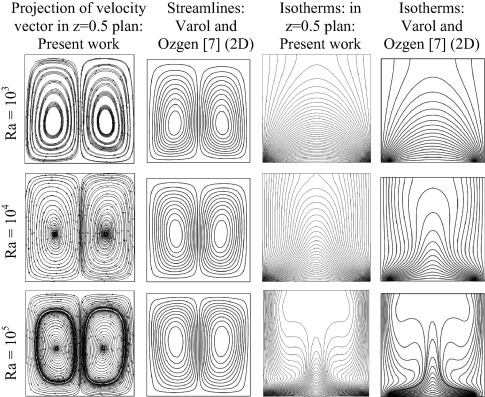Figure 2. Comparison of results of present code with those of Varol and Ozgen (2D) .

## 4. Results and discussion

A computational study is performed in this work is to make three dimensional analysis of natural convection and entropy generation. A computer code was written by using Fortran platform. All results are presented for Ra = 105, Pr = 0.07, and thermal conductivity ratio changes from 0.01 to 100.

Particle trajectories are presented in Fig. 3 for different inclination angles of the fin and Rc = 1.0. This figure gives an idea on particle transport way inside the cavity. As seen from the figures, particle trajectories are continued for θ = 0°. These figures show that two-dimensional solutions are not enough for solution of cubic cavity. Complexity of trajectories is dependent on inclination angle of the fin and it becomes messy behind the fin due to narrow space. Iso-surfaces of temperature for Rc = 1.0 at different inclination angles of the fin are given in Fig. 4. This figure gives an idea on three dimensional distribution of temperature. The distribution of temperature is almost parallel to horizontal walls near the walls. However, they exhibit a parallel distribution to each other near the top side of the fin for θ = 60° and 30°. In case of θ = −30° and θ = −60°, temperature distribution behaves almost as empty cavity due to values of thermal conductivity value. As shown in Fig. 5 the fin is the main factor to manager of the flow and heat transfer inside the cavity. This is clearer from the velocity projections in Fig. 5 at different inclination angles and different thermal conductivity ratios. In case of θ = 60°, the flow is captured behind the fin and dimension of its main eye increases with increase in thermal conductivity due to increase in flow strength. The main flow does not change with thermal conductivity ratio. Similar distribution is observed for θ = 30° and velocity distribution is completely symmetric for θ = 0°. The fin separates the flow two ways in case of θ = −30°. A number of cells are affected from the thermal conductivity and almost a triangular cross-sectional cavity is formed behind the fin for θ = −60° and the flow behaves as almost empty cavity at the remaining part and the main flow is not affected by changing the thermal conductivity ratio.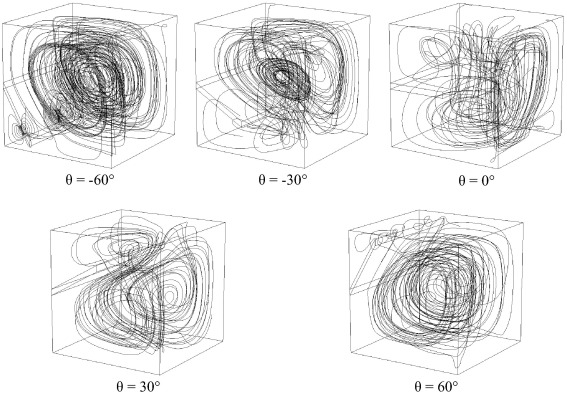Figure 3. Particle trajectories for Rc = 1 at different inclination angles of the fin.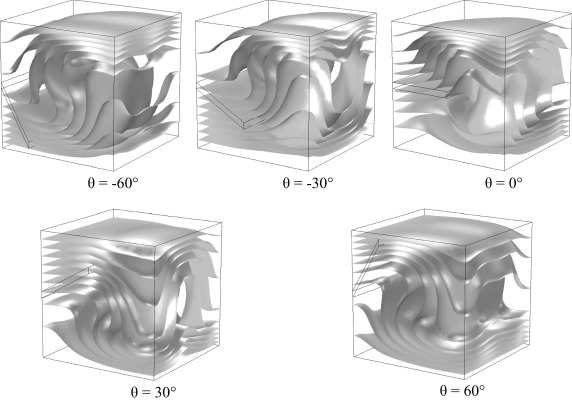Figure 4. Iso-surfaces of temperature for Rc = 1 at different inclination angles of the fin.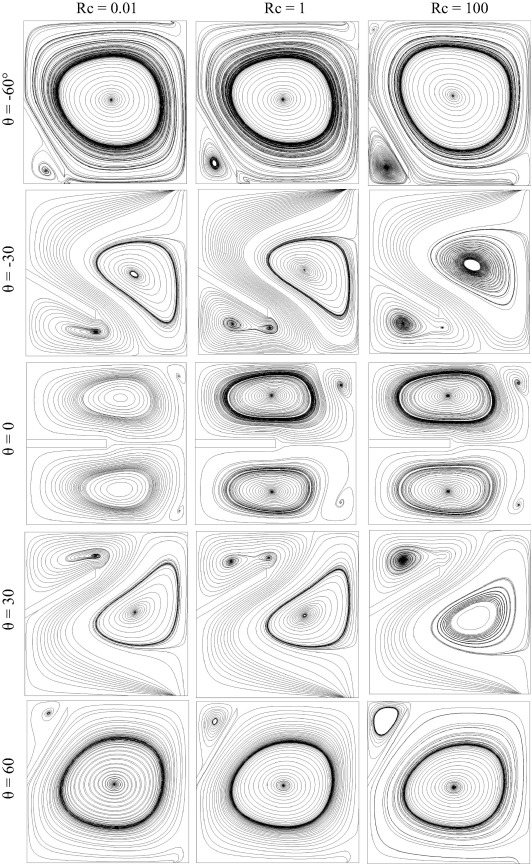Figure 5. Velocity projection in z = 0.5 plan at different inclination angles of the fin and different thermal conductivity ratios.

Fig. 6 illustrates the isotherms for different inclination angles of the fin and thermal conductivity ratio. In case of Rc = 1.0, the fin makes minor effects on temperature distribution inside the cavity. For θ = 60°, isotherms are cumulated on fin at Rc = 0.01 and the fluid behind the fin becomes stagnant due to low conductivity of the fin. This situation is valid for the case of θ = 30°. For the case of high conductivity, inclination angle of the fin becomes an important parameter. For θ = 0°, symmetric distribution is observed for temperature for all values of thermal conductivity due to bottom heating of the cavity.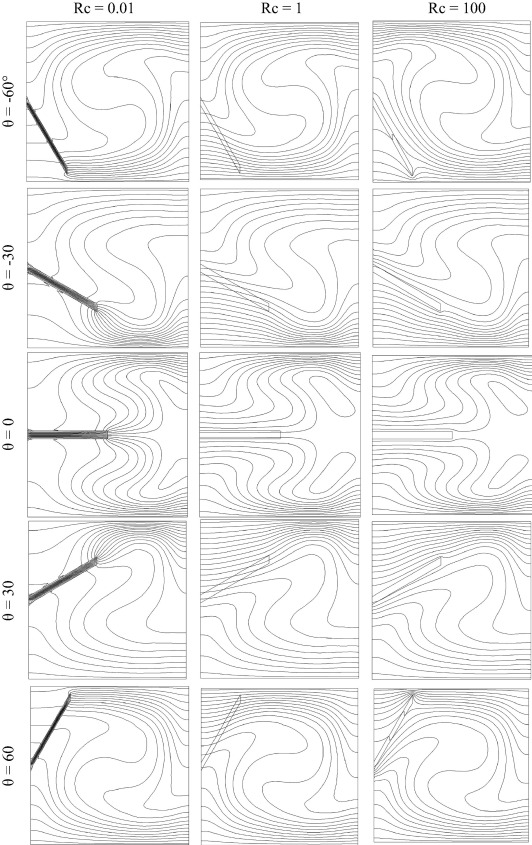Figure 6. Isotherms in z = 0.5 plan at different inclination angles of the fin and different thermal conductivity ratios.

Fig. 7 is plotted to show the local entropy generation due to heat transfer for different inclination angles of the fin and different thermal conductivity ratios. As seen from the figure, inclination angle of the fin plays an important role on distribution of local entropy generation. In case of θ = −60° and Rc = 0.01, local entropy generation contours are accumulated inside the fin due to lower thermal conductivity ratio. For Rc = 1.0, the location of the fin becomes insignificant on entropy generation. However, edge of the fin plays an important role on entropy generation for Rc = 100. Almost symmetric distribution is observed for θ = 0°, and plume like distribution is observed for all values of thermal conductivity ratios due to separation effect of the fin to cavity. For the positive values of inclination angles, the flow inside the cavity is deferred to right top corner and energy is lost at that part mostly.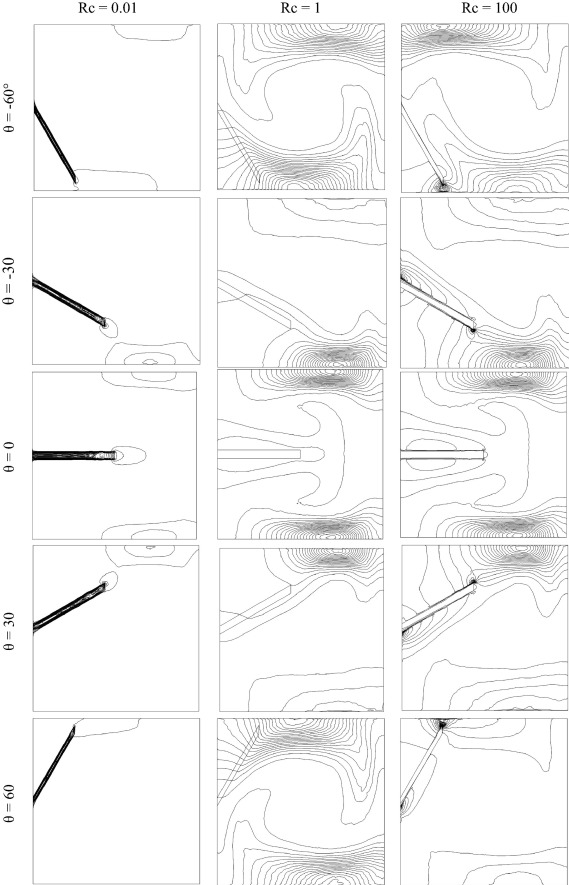Figure 7. Local entropy generation due to heat transfer in z = 0.5 plan at different inclination angles of the fin and different thermal conductivity ratios.

Local entropy generation due to friction is illustrated in Fig. 8. Effects of inclination angle of the fin and thermal conductivity ratio are evaluated in this figure. It can be seen that thermal conductivity ratio is not effective on entropy distribution and contours are cluster middle of the right vertical wall and bottom of the inclined fin for θ = 60°. Bottom edge of the fin affects entropy generation due to moving flow from the bottom and flow separation at that point for θ = 30°. Similar situation is observed for θ = 0° but top and bottom edges of the fin become effective. When the fin turns the negative axis only top edge becomes effective on entropy generation. For θ = −60°, thermal conductivity ratio plays an important role and cumulated entropy contour moves from bottom to edge of the fin while thermal conductivity increases.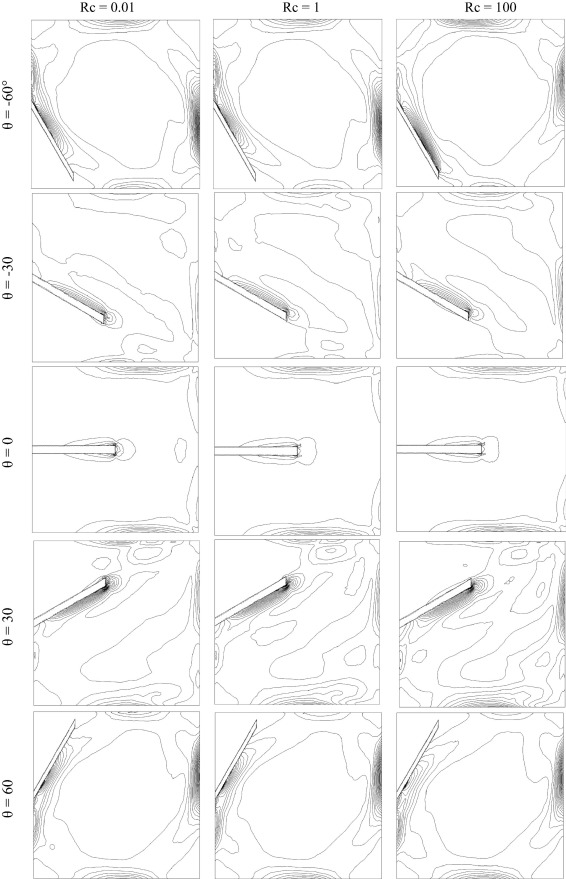Figure 8. Local entropy generation due to friction in z = 0.5 plan at different inclination angles of the fin and different thermal conductivity ratios.

Local total entropy generation is shown in Fig. 9 in z = 0.5 plan for different inclination angles of the fin and different thermal conductivity ratios. The figure shows that edge of the fin becomes effective on entropy generation for all parameters due to flow separation at that point and impinged flow onto the fin. Also, entropy generation changes place to place inside the cavity based on flow distribution and position of the fin. As obtained from the flow and temperature distribution, local entropy distribution shows symmetrical distribution for θ = 0°. The inclination angle of the fin can be a control parameter for energy saving. For example, entropy generation values become stronger near the edge and top side of the cavity for θ = 60 and Rc = 100. Edge effect of the fin becomes minimum for Rc = 1.0 for all values of inclination.Figure 9. Local total entropy generation in z = 0.5 plan at different inclination angles of the fin and different thermal conductivity ratios.

Fig. 10 presents the distribution of local Bejan number which is calculated via Eq. (10). This distribution is given in z = 0.5 plan at different inclination angles of the fin and different thermal conductivity ratios. As indicated earlier that the presence of the fin is a power source for entropy generation, Fig. 11 illustrates the variation of average Nusselt number with the considered parameters of fin angle and thermal conductivity ratio. The average heat transfer shows almost same results for −30° and 30° due to the occurrence of the same effects. In case of Rc = 1.0, a peak is observed. However, higher heat transfer is formed for other inclination angle of the fin and maximum heat transfer is formed for −60 degree at Rc = 100.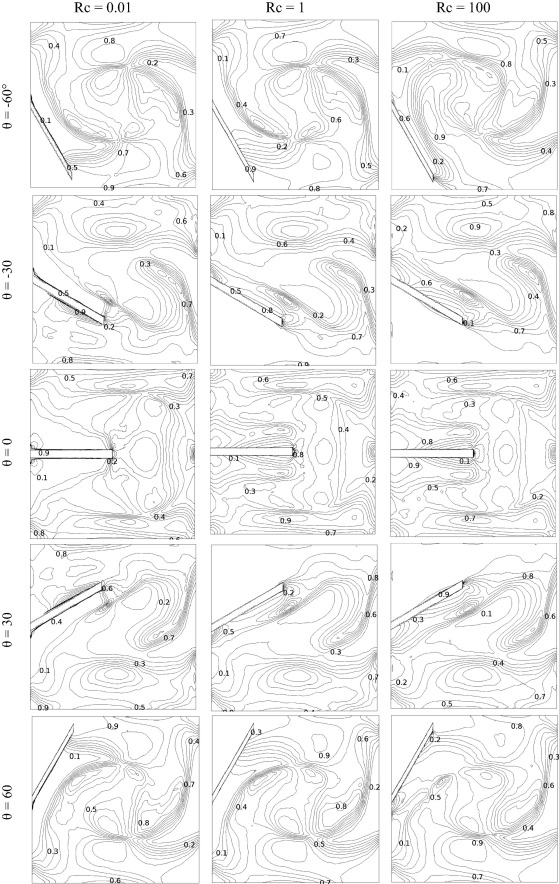Figure 10. Local Bejan number in z = 0.5 plan at different inclination angles of the fin and different thermal conductivity ratios.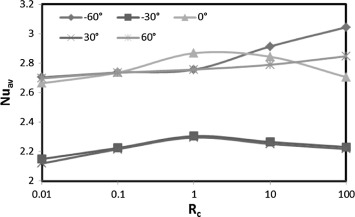Figure 11. Variation of average Nusselt number versus Rc at different inclination angles.

Fig. 12 displays the variation of entropy generation due to heat transfer for different parameters. Sth value is decreased until Rc = 1.0 and it goes constant due to higher value of thermal conductivity ratio. The minimum value is formed for −30° due to motionless flow under the fin. Entropy generation due to friction for different values of thermal conductivity and inclination angle is presented in Fig. 13. The figures show that position of the fin becomes very effective on entropy generation due to friction. Values are decreased with increase in thermal conductivity almost linearly except −60° of the fin angle.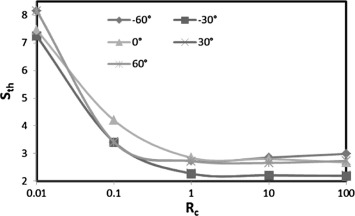Figure 12. Entropy generation due to heat transfer versus Rc at different inclination angles.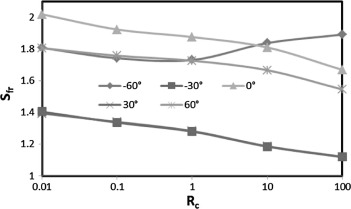Figure 13. Entropy generation due to friction versus Rc at different inclination angles.

Variation of total entropy generation with different inclination angles of the fin and thermal conductivity ratio is presented in Fig. 14. The figure gives that these two parameters are good control parameter or optimization parameter for energy consumption. In other words, maximization or minimization is possible inside the cavity by using fin. As seen from the figure, total entropy generation decreases up to Rc = 1.0 and it becomes constant after these value. It is noted that the fin is more conductive for higher value of Rc. The highest entropy generation occurs for −30 and the lowest value is observed for 0°. For all values there is a minimum point around Rc = 1.0.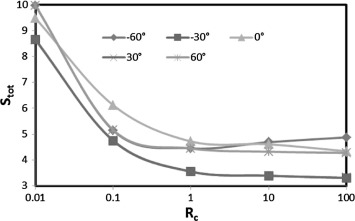Figure 14. Total entropy generation versus Rc at different inclination angles.

Fig. 15 illustrates the variation of Bejan number versus Rc for different inclination angles of the conductive fin. As well known from the literature that Bejan number gives the ratio of heat transfer and fluid friction, thus, as seen from the figure, Bejan number decreases with thermal conductivity ratio till Rc = 1.0 and it becomes almost constant for all values of thermal conductivity ratio.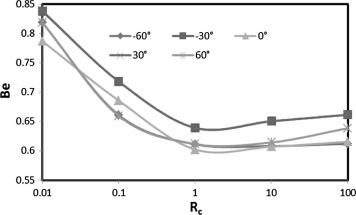Figure 15. Variation of Bejan number versus Rc at different inclination angle.

## 5. Conclusions

A three dimensional computational study has been performed in this work in an inclined fin located cavity to obtain heat transfer, temperature distribution, fluid flow and entropy generation. In this work, parameters are taken as Ra = 105, Pr = 0.7, Rc   (conductivities ratio) changes from 0.01 to 100 and irreversibility coefficient is taken as ${\textstyle \varphi ={10}^{-5}}$. Firstly, three dimensional solution in a cubic cavity brings some advantages according to two dimensional solution to see the effects of heat and fluid flow in different directions. The main effective parameter on heat and fluid flow is the inclination angle of the fin. There is symmetrical distribution on temperature distribution and flow field in a cavity when θ = 0°. Edge of the fin is extremely effective on entropy generation and Bejan number for all studied parameters. Entropy generation distribution is also obtained as symmetric for all values of thermal conductivity ratio. But higher values of thermal conductivity ratio (Rc ⩾ 1) are not effective on entropy generation. For Rc = 1, there is a maximum or minimum value on heat transfer according to inclination angle of the fin. The highest heat transfer is seen for the −60° of the fin angle and Rc = 100. It is an interesting result that heat transfer gives same results for −30° and 30° of the fin angle. Both entropy generation and heat transfer become higher for positive values of inclination angle. Entropy generation due to heat transfer becomes dominant onto friction one.

### Document informationPublished on 12/04/17

Licence: Other

### Document Score0

Views 32
Recommendations 0